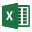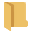Join one of our upcoming courses taking place around the world! Explore now

# Overview of Automate from Excel

Automate from Excel can quickly generate results from inputs read in from Excel files. Numerical input data is read into the UnWedge computation engine from a formatted Excel file and results are generated for each defined case without the need to create a separate UnWedge model file for each.

WARNING: The Automate from Excel feature is dedicated to advanced users who are familiar with the user interface, inputs, and assumptions of the program. Invalid input data will generate invalid results.

To access the Automate from Excel feature of UnWedge:

1. Select File > Automate Compute > Automate from Excelfrom the menu.
2. The Automated Compute dialog will appear allowing you to:
• Add files to the File Queue for computing
• Optionally select if you -want to save each computed case as a model file by checking the Save As UnWedge File(s) checkbox
• See the progress of Completed Files
• See if any issues occurred during reading, computing, or writing under Warnings

## Input File

The formatting of the input Excel files is specific to the program and Analysis Type set in the Project Settings dialog.

Formatted Excel templates are provided for you and can be accessed by selecting Deterministic Excel Template, or Probabilistic Excel Template from the File > Automate Compute sub-menu. Each UnWedge Excel template is formatted specifically for use with the corresponding UnWedge analyses.

Automate Compute several Deterministic, and/or Probabilistic Analysis cases using saved Excel files created from UnWedge Excel templates.

To add Excel files to the File Queue:

• Select the Openbutton to choose individual model files.
Only use UnWedge Excel templates provided. Ensure that the correct template type (i.e., Deterministic Excel Template, or Probabilistic Excel Template) is used, which corresponds with the Analysis Type (i.e., Deterministic, or Probabilistic) set in UnWedge Project Settings.

For more information, see the Automate from Excel Deterministic Analysis, and Probabilistic Analysis topics.

## Output File

When Automate Compute is run, a directory by the same name as the input model file will be created in the same directory as the input model file.

The results of each model file is output to Excel (.xlsx) format. At least one "Results" file is generated per case computed.

In a Deterministic Analysis, one "Results" file per Excel input file is generated.

In a Probabilistic Analysis where more than one tunnel can be considered, one "Results" file per input case is generated. The resulting file is formatted to write one analysis per row.

The output file contains 12 sheets:

• General
• Field Stress
• One sheet per wedge (8 possible Perimeter Wedges, 2 possible End Wedges)

### GENERAL DATA

The General sheet contains the following global data:

• Tunnel ID
• Tunnel Trend
• Tunnel Plunge
• Tunnel Length
• Design FS
• Rock Unit Weight
• Water Unit Weight
• Seismic Coeff.
• Seismic Coeff. X/North
• Seismic Coeff. Y/East
• Seismic Coeff. Z/Down
• Seismic Trend
• Seismic Plunge

### FIELD STRESS DATA

The Field Stress sheet contains the following global data:

• Tunnel ID
• Sigma 1
• Sigma 1 Trend
• Sigma 1 Plunge
• Sigma 2
• Sigma 3
• Sigma 3 Trend
• Sigma 3 Trend
• Ground Surface Elev.
• Major Hor. Stress Tend
• Major Hor. Stress Ratio
• Minor Hor. Stress Ratio
• Young's Modulus
• Poisson Ratio
• Num Elements
• Num Joint Sample Pts.

### WEDGE DATA

Each Wedge sheet contains the following wedge-specific data:

• Tunnel ID
• Wedge Name and Number
• Factor of Safety
• Wedge Volume
• Wedge Weight
• Wedge z-Length
• Excavation Face Area
• Support Pressure
• Resisting Force
• Driving Force
• Shear Force
• Apex Height
• Apex Angle
• Scaling Parameter
• Scaled By
• Failure Mode
• Sliding Joint Orientations
• Sliding Direction (East, North, Up)
• Sliding Direction (Trend, Plunge)
• Joint Codes, for all three joints
• Joint Dip, for all three joints
• Joint Dip Direction, for all three joints
• Friction Angle, for all three joints
• Cohesion, for all three joints
• Tensile Strength, for all three joints
• JRC, for all three joints
• JCS, for all three joints
• PhiR, for all three joints
• Power Curve A, for all three joints
• Power Curve B, for all three joints
• Power Curve C, for all three joints
• Power Curve D, for all three joints
• Waviness, for all three joints
• Water Pressure, for all three joints
• Water Elevation, for all three joints
• Joint Area, for all three joints
• Joint Persistence, for all three joints
• Joint Trace Length, for all three joints
• Joint Normal Force, for all three joints
• Joint Water Force, for all three joints
• Joint Shear Strength, for all three joints

#### Notes:

• If no valid wedge is formed, Factor of Safety = N/A and any computed values will also be N/A.
• The applicability of some computed values depends on the geometry, strength, force, and water options selected.

## Model File

If Save As UnWedge File(s) checkbox is selected, UnWedge will create a model file per case computed, based on the Excel Template inputs. The model files are placed in a directory by the same name as the input model file, in the same directory as the input model file (same as where the results files are placed).

## Error Log

An Error Log text file (Automate Compute Error Log.txt) is generated for each input Excel file after Automate Compute.

The Error Log reports the total number of errors found (if any), the cell(s) where the error occurred, and the reason why an error occurred. An error will occur if a required parameter cell:

• Is Blank, or
• Has a value outside the valid range
The Error Log should always be inspected to ensure "No errors found".